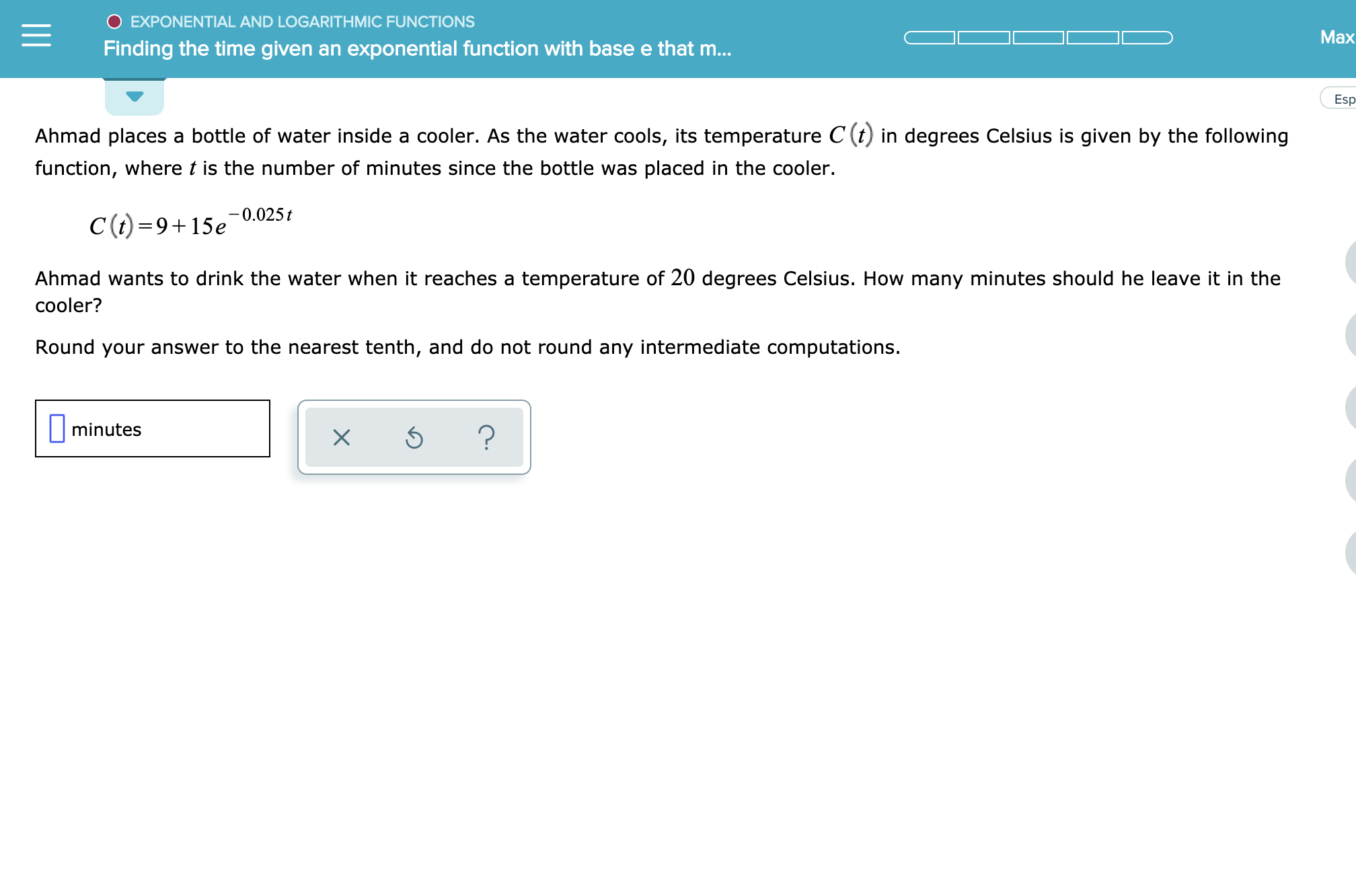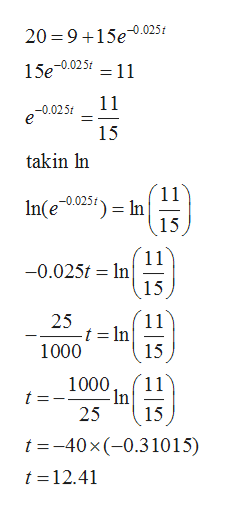# O EXPONENTIAL AND LOGARITHMIC FUNCTIONSMaxFinding the time given an exponential function with base e that m...Esp-Ahmad places a bottle of water inside a cooler. As the water cools, its temperature C (t) in degrees Celsius is given by the followingfunction, where t is the number of minutes since the bottle was placed in the cooler.C(t)=915e 0.025tAhmad wants to drink the water when it reaches a temperature of 20 degrees Celsius. How many minutes should he leave it in thecooler?Round your answer to the nearest tenth, and do not round any intermediate computations.minutes?X

Question
3 views

See attachmenthelp_outlineImage TranscriptioncloseO EXPONENTIAL AND LOGARITHMIC FUNCTIONS Max Finding the time given an exponential function with base e that m... Esp- Ahmad places a bottle of water inside a cooler. As the water cools, its temperature C (t) in degrees Celsius is given by the following function, where t is the number of minutes since the bottle was placed in the cooler. C(t)=915e 0.025t Ahmad wants to drink the water when it reaches a temperature of 20 degrees Celsius. How many minutes should he leave it in the cooler? Round your answer to the nearest tenth, and do not round any intermediate computations. minutes ? X fullscreen
check_circle

Step 1

The given temperature function C(t) is

Step 2

So at C(t)=20 degr...help_outlineImage Transcriptionclose20 915e-0.025 15e-0.025t 11 11 -0.025 15 takin In 11 In 15 In(e 025) In 15 -0.025 1 25 t = In 1000 11 15 1000 11 In 25 t 15 t -40 x(-0.31015) t -12.41 fullscreen

### Want to see the full answer?

See Solution

#### Want to see this answer and more?

Solutions are written by subject experts who are available 24/7. Questions are typically answered within 1 hour.*

See Solution
*Response times may vary by subject and question.
Tagged in

### Other